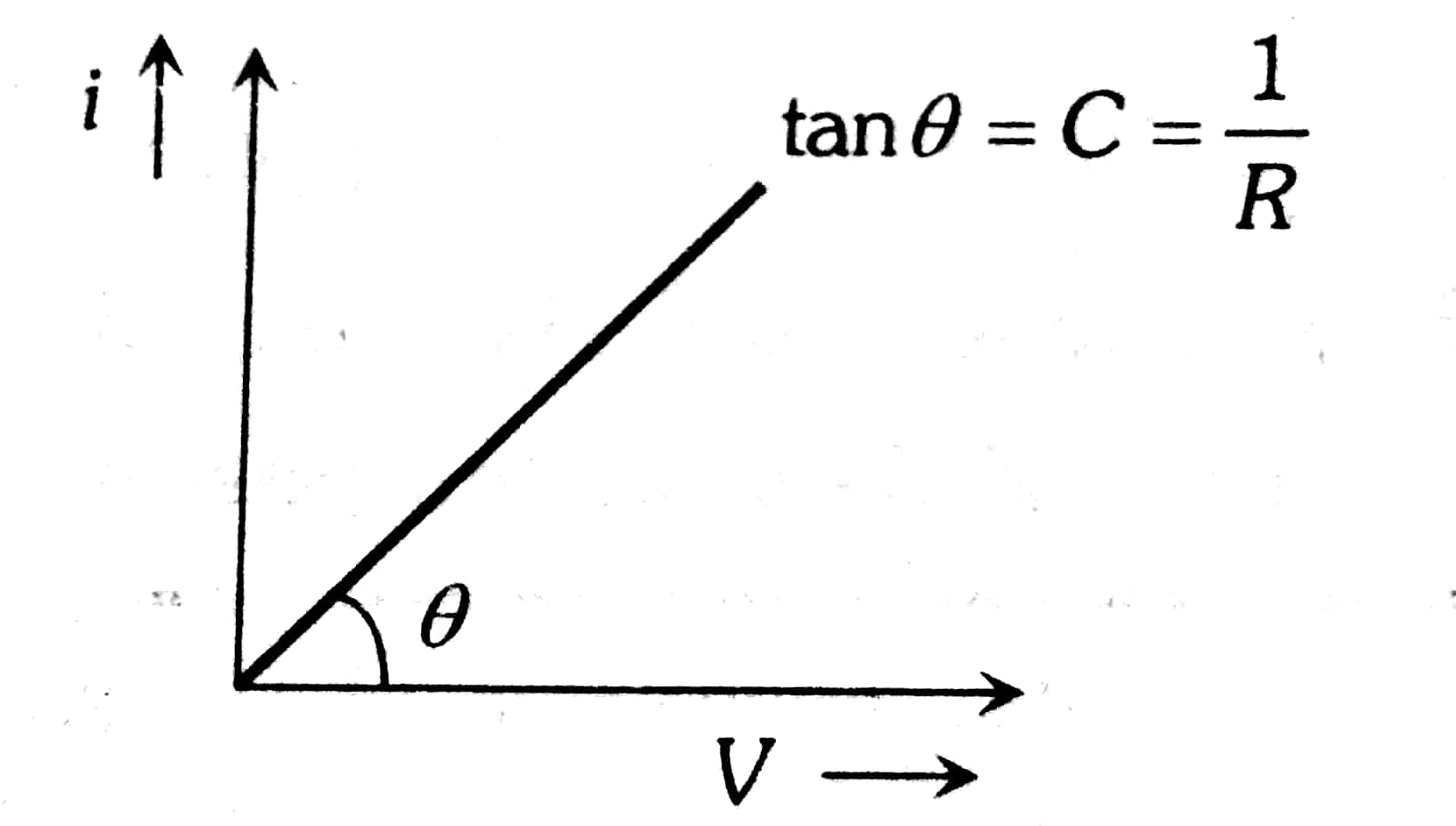# A copper wire of conductance 72 Ω-1 is melted and redrawn to thrice its original length. Find the Conductance of stretched wire. Option 1) 6 Ω-1 Option 2) 8 Ω-1 Option 3) 9 Ω-1 Option 4) 12 Ω-1

P Plabita

As we learnt

Conductance -

Conductance

- whereinSince volume of the wire does not change.

Þ l1A1 = l2 A2  where l­1­  and A1 are the initial length and cross-section of the wire, and l2 and A2 are the final length and cross-section.

Þ

C1 = A1/ρL1   and     C2 = A2/ ρ L1

Þ           C1/C2 = L22/L21    = 9

Þ C2= C1/9 = 8 Ω-1

Option 1)

6 Ω-1

Option 2)

8 Ω-1

Option 3)

9 Ω-1

Option 4)

12 Ω-1

Exams
Articles
Questions